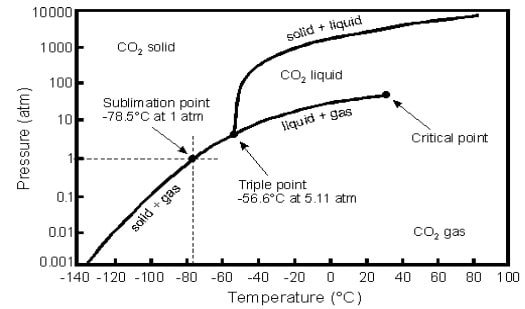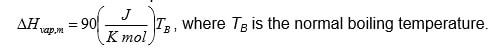# AES1300 Properties of Hydrocarbons and Oilfield Fluids

1. Phase diagram for pure component:Fig.1 Pressure-Temperature diagram for CO2

1. We perform an experiment on CO2 in a closed container (V = const) with the following steps:
• The experiment starts at T = 20oC and p = 200 bars
• We drop the pressure in container down to p = 10 bar until T equals -60 o
• Next, keeping the same T, we increase p up to 200 bars again
• We heat container up to T = 20oC again

Describe all states of CO2 at each stage of the experiment. Describe also the assumptions you made in order to choose a particular connection path. Use the diagram and report (approximate) changes in p and T connected with the state of CO2 during each stage of experiment. Are we going to arrive to the same state by the end of the experiment? Explain why.

1. Recall the equation of Clausius and the Clausius-Clapeyron describing the vapor pressure curve. Give the Clausius equation before integration as dp/dT and the Clausius-Clapeyron equation after the integration a p = f(T). Which assumptions are made to allow the integration?
1. The molar enthalpy of vaporization can be described by the so-called Trouton’s law. For a certain component Trouton’s law can be written as:Determine the molar enthalpy of vaporization in J/mol if the component boils at 1200C at a pressure of 1 bar.

1. Give the equation to describe the vapor pressure curve for this component (based on the Clausius-Clapeyron equation). Plot the vapor pressure in bars as function of the temperature in the range from 10 to 150o Use MATLAB.
1. Determine for this component the boiling point (temperature) at 50 bars (assuming that this pressure is lower than the critical pressure).
1. Deliver short report of the MATLAB task in Section 1
1. A hydrocarbon mixture having the composition given in table 1 is submitted to a petroleum laboratory for analysis. The mixture is maintained at a pressure of 1 atmosphere and a temperature of 60 0
• Write down all possible structural formulae of the compounds. Explain why you choose particular structure.
• Which components are isomers?
• Would you expect the hydrocarbon mixture to be a liquid or a gas phase? Explain.
• How much water would be produced upon burning 250 g of the hydrocarbon mixture in the presence of oxygen?

Table 1

 Hydrocarbon Weight % Boiling Freezing point At 1 atm [K] Molecular weight [g mol-1] temperature at 1 atm [K] Methane 25.7 111.66 90.69 16.043 Ethane 6.4 184.55 90.35 30.07 Propane 5.3 231.02 85.47 44.097 Isobutane 0.2 261.34 113.54 58.123 n-Butane 2.4 272.66 134.79 58.123 Benzene 9.8 353.24 278.68 78.114 Toluene 8.5 383.79 178.16 92.141 Ethylbenzene 9.2 409.36 178.18 106.167 o-Xylenes 11.3 417.59 247.97 106.167 1,2,4-Trimethylbenzene 9.9 442.49 229.35 120.194 CO2 11.3 -. 216.58 44.01
1. Upon lowering the temperature of 500 g of the above mentioned hydrocarbon mixture below 7 0C we obtain a liquid residue of the aromatic compounds while the non-aromatic hydrocarbons remain essentially in the gaseous phase.
• Determine the composition of the gas obtained and then the composition of the liquid residue. Compositions are required in weight percent (wt%).
• How much carbon dioxide would be produced by combustion of the gas from exercise a?
1. In 4-component system representing typical reservoir fluid, at most 3 phases can co-exist simultaneously (aqueous and liquid and vapour hydrocarbon). Describe the dimensions (point, line, area, volume) of all possible combination of phases.